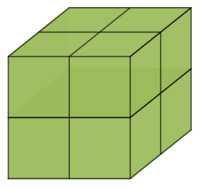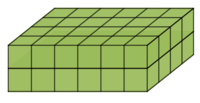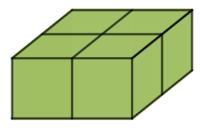# Volumes with Fractional Edge Lengths

Alignments to Content Standards: 6.G.A.2

Make sure you have plenty of snap cubes.

1. A dubsnap is a length equal to two snap cube edges. Build a cube using 8 snap cubes of one color. Call this a dubsnap cube, with side length equal to 1 dubsnap, so it has a volume of $1\times 1\times 1=1$ cubic dubsnap.
1. How long (in dubsnaps) are the side lengths of a single snap cube?
2. What is the volume of a single snap cube, in cubic dubsnaps?
2. Build a rectangular solid that is 2 snap cubes by 4 snap cubes by 6 snap cubes. What is the volume of this solid in cubic dubsnaps?
1. What are the side-lengths (in dubsnaps) of a solid that is 1 snap cube by 4 snap cubes by 6 snap cubes? What is its volume in cubic dubsnaps?
2. What are the side-lengths (in dubsnaps) of a solid that is 2 snap cubes by 2 snap cubes by 6 snap cubes? What is its volume in cubic dubsnaps?
3. What are the side-lengths (in dubsnaps) of a solid that is 2 snap cubes by 2 snap cubes by 3 snap cubes? What is its volume in cubic dubsnaps?
3. Build a solid that has a volume of $\frac12$ cubic dubsnap.
4. Build a solid that has a volume of $\frac58$ cubic dubsnap.

## IM Commentary

The purpose of this task is to introduce students to fractional units for volume. This task provides opportunities to

• extend the formula $V=lwh$ for non-whole number side lengths;
• practice fraction multiplication and verify the result geometrically;
• highlight the fact that a volume can be a numerically smaller number than the lengths of its sides.

A note about "plenty of snap cubes" for planning: the largest rectangular solid that a student would build for this task consists of 48 snap cubes. It would be appropriate for students to work in pairs for this task, so you don't necessarily need 48 snap cubes for every student. It may be helpful, ahead of time, to cut up and provide students with lengths of straws that are each the length of two snap cube edges (one dubsnap).

We chose the word "dubsnaps" in place of "unit" to avoid any misreadings, since "unit" often implies "one." But it would be mathematically fine, if desired, to replace all instances of "dubsnap" in the task with "unit" (or even with a new word that the class likes better.)

We suggest students be given time to work and make sense of the task with intermittent pauses for whole-class clarification and discussion. It will be necessary for students to attend to precision (MP6) as they communicate about the problem and their solutions, because if they neglect units of measure while speaking, this is likely to lead to confusion. Once students have had a chance to struggle a bit on their own, the teacher should listen for the language students are using and encourage precision where needed.

As a part of whole-class discussion, the teacher should highlight the geometric interpretation of the volume alongside the result of using a formula like $V=lwh$ that students should know from previous work with whole numbers. For example, part (a)(ii) asks about the volume of a single snap cube. A single snap cube is geometrically $\frac18$ of a dubsnap cube, but also $\frac18$ is the product $\frac12 \times \frac12 \times \frac12$.

One idea might be to preface this task with discussion about the statement, "Always, Sometimes, or Never: The volume of a rectangular prism is a larger number than any of its side lengths." Many students may initially conjecture "always" and give some examples, and then the statement could be revisited after this task.

We highly recommend that students work with physical snap cubes. This Desmos sketch is provided as a resource, perhaps for demonstration purposes. (Created by Nathan Kraft.)

## Solution

1. The dubsnap cube should look like:1. The side length of a single snap cube is $\frac12$ dubsnap.
2. The volume of a single snap cube is $\frac12\times\frac12\times\frac12=\frac18$ cubic dubsnaps.
2. The rectangular solid should look like:Its volume is 6 cubic dubsnaps.
1. The side-lengths are $\frac12$ dubsnap (1 snap cube) by 2 dubsnap (4 snap cubes) by 3 dubsnap (6 snap cubes). The volume is 3 cubic dubsnaps. This can be seen by disassembling the solid into 3 cubes made of 6 snap cubes each.
2. The side-lengths are 1 dubsnap (2 snap cubes) by 1 dubsnap (2 snap cubes) by 3 dubsnaps (6 snap cubes). The volume is 3 cubic dubsnaps. This can be seen by disassembling the solid into 3 cubes made of 6 snap cubes each.
3. The side-lengths are 1 dubsnap (2 snap cubes) by 1 dubsnap (2 snap cubes) by $1\frac12$ dubsnaps (3 snap cubes). The volume is $1\frac12$ cubic dubsnaps. This can bee seen by putting two copies together to get a single cubic dubsnap.
3. Answers may vary and do not have to be rectangular solids. This solid has a volume of $\frac12$ cubic dubsnap:4. Answers may vary and do not have to be rectangular solids. This solid has a volume of $\frac58$ cubic dubsnap: